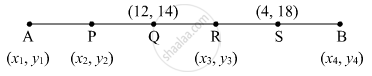Share

# The Line Segment Ab is Divided into Five Congruent Parts at P, Q, R and S Such that A–P–Q–R–S–B. If Point Q(12, 14) and S(4, 18) Are Given Find the Coordinates of A, P, R, B. - Geometry

#### Question

The line segment AB is divided into five congruent parts at P, Q, R and S such that A–P–Q–R–S–B. If point Q(12, 14) and S(4, 18) are given find the coordinates of A, P, R, B.

#### SolutionLet the coordinates be

$A\left( x_1 , y_1 \right)$ $P\left( x_2 , y_2 \right)$, $R\left( x_3 , y_3 \right)$ and B $\left( x_4 , y_4 \right)$.
QR = RS
$R\left( x_3 , y_3 \right) = \left( \frac{12 + 4}{2}, \frac{14 + 18}{2} \right) = \left( 8, 16 \right)$
RS = SB

$B\left( x_4 , y_4 \right) = \frac{8 + x_4}{2} = 4, \frac{16 + y_4}{2} = 18$

$\Rightarrow 8 + x_4 = 8, y_4 = 36 - 16$

$\Rightarrow x_4 = 0, y_4 = 20$

$B\left( x_4 , y_4 \right) = \left( 0, 20 \right)$

$\frac{AQ}{QB} = \frac{AP + PQ}{QR + RS + SB} = \frac{AP + AP}{AP + AP + AP} = \frac{2}{3}$

$\frac{2 \times 0 + 3 \times x_1}{2 + 3} = 12, \frac{2 \times 20 + 3 \times y_1}{2 + 3} = 14$

$\Rightarrow 3 x_1 = 60, 40 + 3 y_1 = 14$

$\Rightarrow x_1 = 20, y_1 = 10$

$A\left( x_1 , y_1 \right)$ = (20, 10)
AP = PQ

$\frac{20 + 12}{2} = x_2 , \frac{10 + 14}{2} = y_2$

$\Rightarrow \frac{32}{2} = x_2 , \frac{24}{2} = y_2$

$\Rightarrow x_2 = 16, y_2 = 12$

Is there an error in this question or solution?